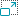To edit an equation:

1.Open the equation editing window in one of the following ways:

Select the equation by clicking the left mouse button. On the Toolbar, under the Equation section, clickEdit.

Select the equation by right-clicking it. Run the Edit Equation context menu command.

Select the equation by double-clicking it with the mouse.

2.In the Equation window, in the LaTeX Expression box, edit the equation.

3.Click ОК.

Was the material useful?
Yes
No
Users found this material useful: 0 из 0